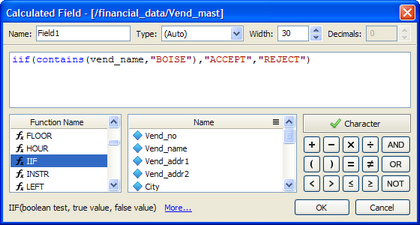﻿ Using the Formula Builder
 Using the Formula Builder

Working with Formulas > Using the Formula Builder

The formula builder is used in a variety of data operations, such as creating calculated fields, filtering your data or copying records to a new table.  It is used for creating formulas as well as viewing and editing existing formulas.The formula builder has four main parts:

 • Formula Box: This is the place where you enter your formula.  It can be entered either typing directly or by clicking on the items available in the builder and must follow the rules for creating formulas.
 • Function Names: The functions available for building formulas.  You may double-click on a function and it will appear in the formula box.  Also, note that if you hover over the function, its syntax will appear in a tooltip.  Also, when you click on a function, its syntax appears at the bottom left of the formula builder.  For further help, click the "More..." link, which will open a help web page for the function.
 • Field Names: The fields available within your data set for building formulas.  You may double-click on a field and it will appear in the formula box.
 • Operators: The mathematical operators available for building formulas.  You may double-click on an operator and it will appear in the formula box.

To use the formula builder, do the following:

 1 Enter the formula, either by typing directly into the formula box or by double-clicking on the functions, etc. to make them appear in the formula box.
 2 Check that the formula validation indicator says "Valid" (green check mark) and click the OK button.  If the status indicator says "Invalid" (red "X"), it means that your formula is not correct and needs to be adjusted.

NOTE:  The formula validation indicator is disabled for external data tables so that native database expressions can be entered in the formula builder and run against the external database.

Finally, there are two other items in the formula builder to help guide your experience:

 1 Function tool tips.  This feature is found when you type in a valid function name in the expression builder. A tool tip will pop up showing you the correct syntax for the function you are about to use.
 2 Formula colors.  Color-coding occurs when you type an expression into the expression builder. For example, if you type in a valid function, the text of the function will turn blue.